# Multivariable Calculus: Higher-Dimensional Differentiation

Can someone help me with what happened after changing w2? Also I am not able to understand how the epsilon 1 times epsilon 2 term came in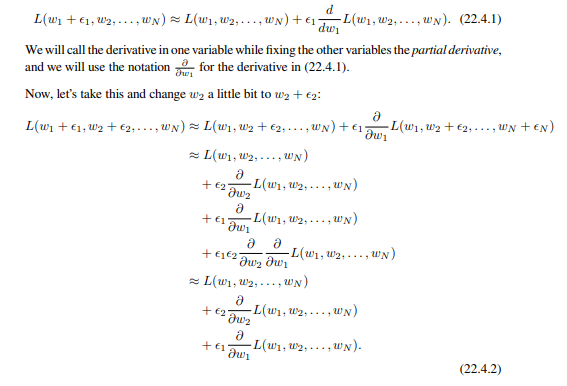So you know that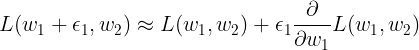Now, if we also modify w2 to be w2 + epsilon_2, need to apply the change not only to the first term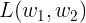But also to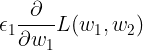See this example:
If we set W_1 = w_1 + epsilon_1, and we apply the rule for w_2, we get: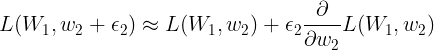But we know that: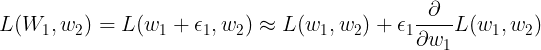So if we substitute it inside our previous equation we indeed get the epsilon_1 * epsilon_2 term that you see in the book.

1 Like

Thank you very much Brian! Its clear now!1 Like